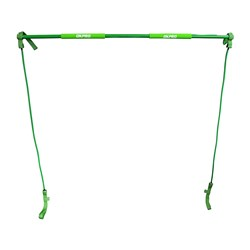# General Fitness

Grid List
\$72.55
Increase value Decrease value
\$38.50
Increase value Decrease value
\$34.99
Increase value Decrease value
PTP Ab Sculpt
In Stock
\$50.00
Increase value Decrease value
\$32.00
Increase value Decrease value
\$39.95
Increase value Decrease value
\$154.00
Increase value Decrease value
\$39.95
Increase value Decrease value
\$329.00
Increase value Decrease value
\$349.00
Increase value Decrease value
\$44.00
Increase value Decrease value
In Stock
\$42.50
Increase value Decrease value
\$129.90
Increase value Decrease value
\$129.90
Increase value Decrease value
\$50.00
Increase value Decrease value
\$9.90
Increase value Decrease value
\$99.99
Increase value Decrease value
\$12.99
Increase value Decrease value
\$79.00
Increase value Decrease value
\$14.99
Increase value Decrease value\$16.50
Increase value Decrease value
\$79.00
Increase value Decrease value
Pro-Stretch Plus
In Stock
\$80.30
Increase value Decrease value
\$199.65
Increase value Decrease value
From
Increase value Decrease value
From
Increase value Decrease value
From
Increase value Decrease value
From
Increase value Decrease value
From
Increase value Decrease value
Grid List
##### Products to compare:
Comparing Products